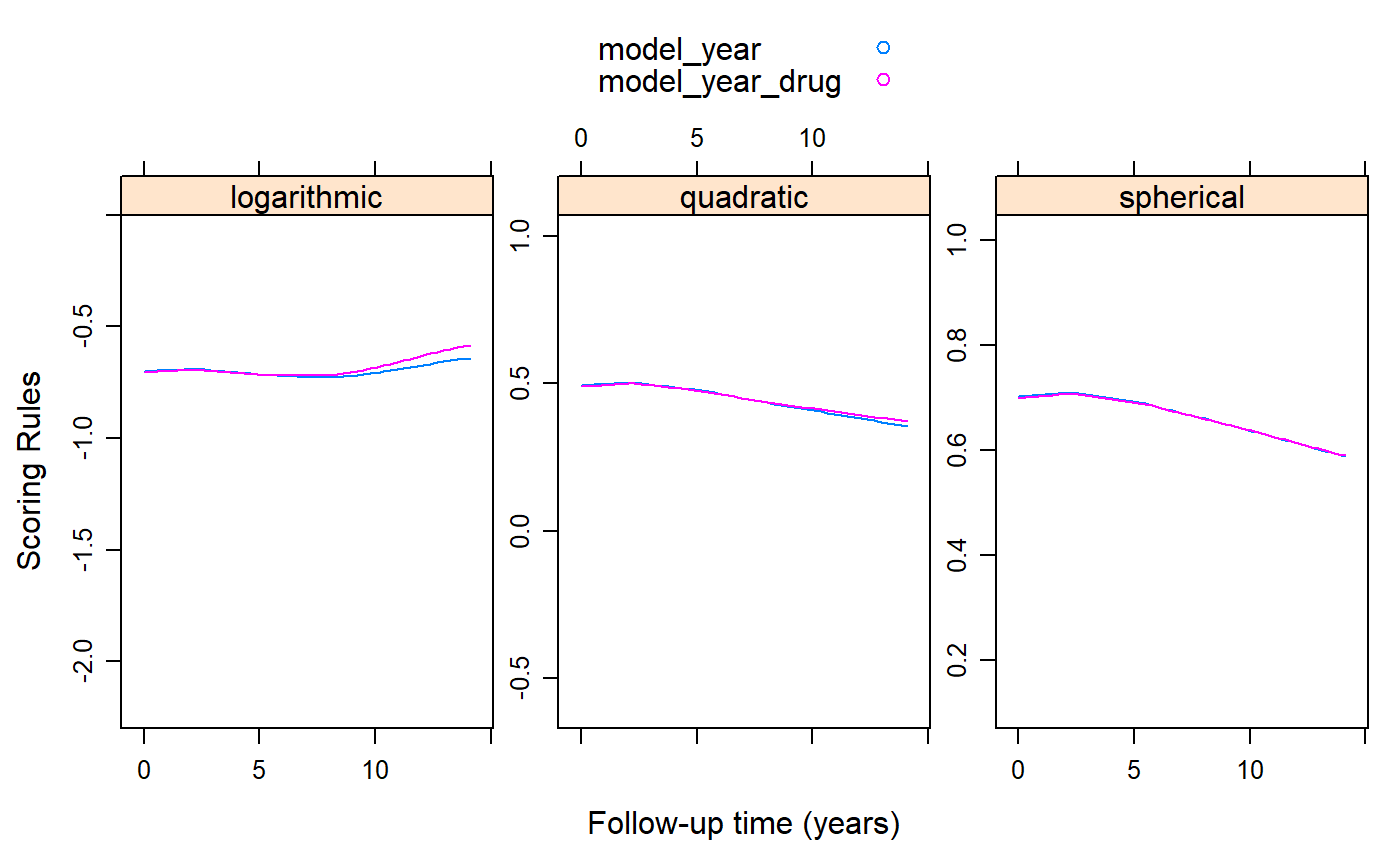Calculates the logarithmic, quadratic/Brier and spherical scoring rules based on generalized estimation equations.

cv_gee(object, rule = c("all", "quadratic", "logarithmic", "spherical"),
max_count = 500, K = 5L, M = 10L, seed = 1L, return_data = FALSE)

## Arguments

object an object inheriting from class "geeglm" of the geepack. character string indicating the type of scoring rule to be used. numeric scalar or vector denoting the maximum count up to which to calculate probabilities; this is relevant for count response data. numeric scalar indicating the number of folds used in the cross-validation procedure. numeric scalar denoting how many times the split of the data in K folds will be performed. The reported scoring rules values are the average over the M replicates. numeric scalre providing the seed used in the procedure. logical; if TRUE the values of the scoring rules are ruturned as extra columns of the data behind object.

## Value

A list or a data.frame with elements or (extra) columns the values of the logarithmic, quadratic and spherical scoring rules calculated based on the GEE object.

Carvalho, A. (2016). An overview of applications of proper scoring rules. Decision Analysis 13, 223-242. doi:10.1287/deca.2016.0337

Liang, K.Y. and Zeger, S.L. (1986). Longitudinal data analysis using generalized linear models. Biometrika 73, 13-22. doi:10.1093/biomet/73.1.13

## Examples

library("geepack")
library("lattice")

pbc2$serBilirD <- as.numeric(pbc2$serBilir > 1.2)

fm1 <- geeglm(serBilirD ~ year, family = binomial(), data = pbc2,
id = id, corstr = "exchangeable")

fm2 <- geeglm(serBilirD ~ year * drug, family = binomial(), data = pbc2,
id = id, corstr = "exchangeable")

plot_data <- cv_gee(fm1, return_data = TRUE, M = 5)
plot_data$model_year <- plot_data$.score
plot_data\$model_year_drug <- unlist(cv_gee(fm2, M = 5))

xyplot(model_year + model_year_drug ~ year | .rule, data = plot_data,
type = "smooth", auto.key = TRUE, layout = c(3, 1),
scales = list(y = list(relation = "free")),
xlab = "Follow-up time (years)", ylab = "Scoring Rules")